Filters
Size

# Men's White T Shirts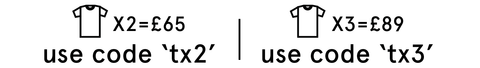Filters

Selected Filters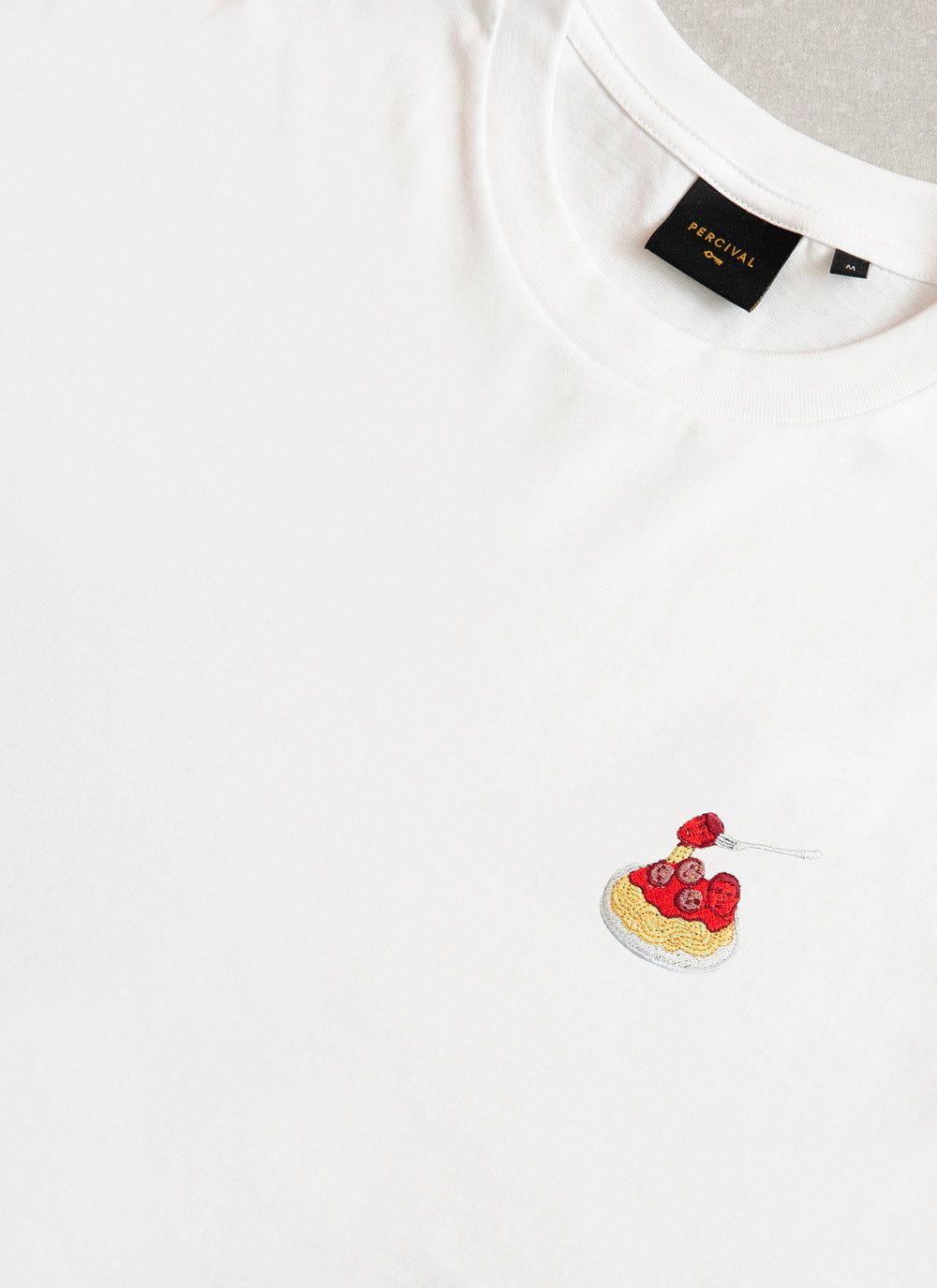• XS
• S
• M
• L
• XL
• XXL
• Select Size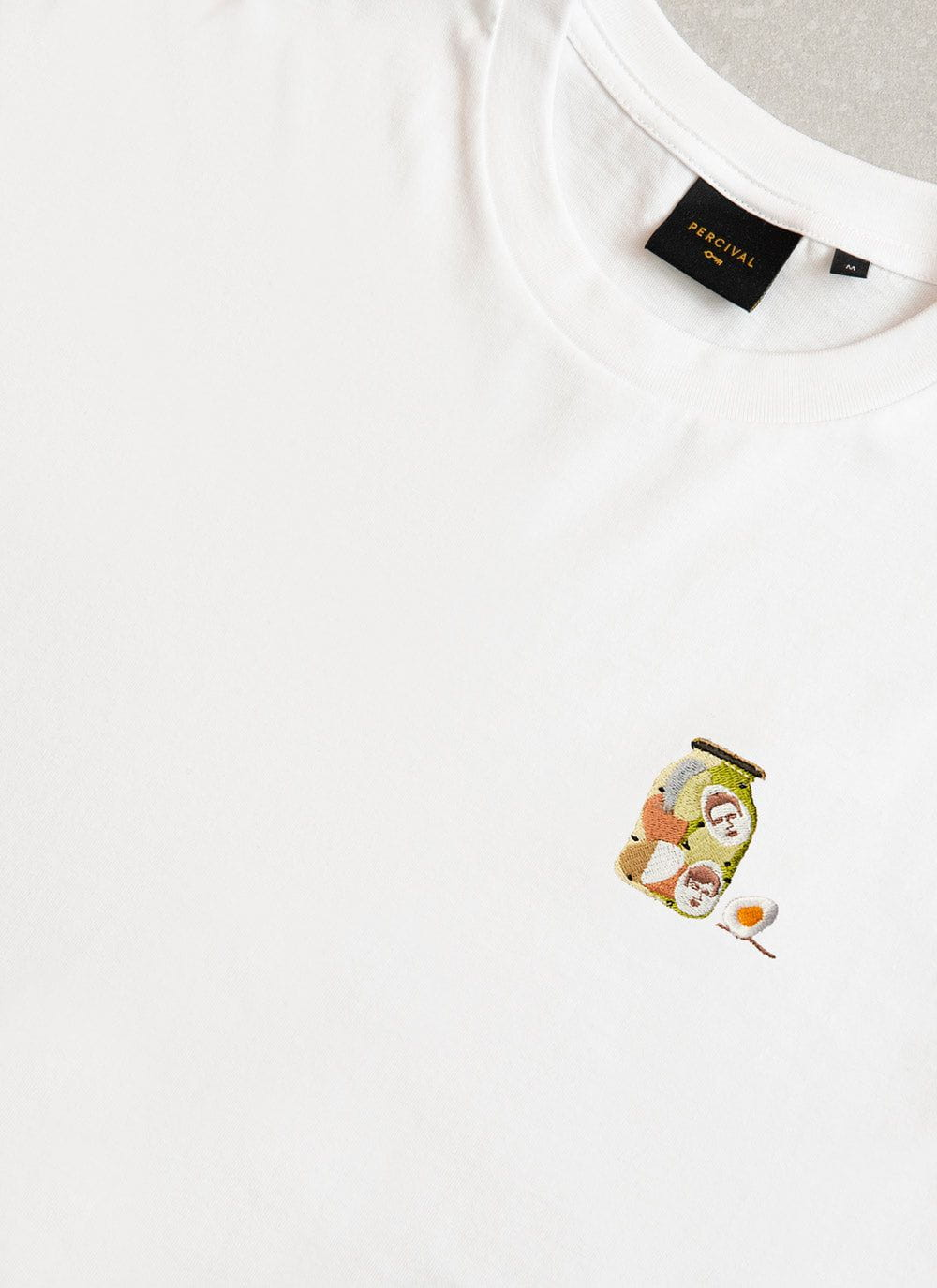• XS
• S
• M
• L
• XL
• XXL
• Select Size• XS
• S
• M
• L
• XL
• XXL
• Select Size• XS
• S
• M
• L
• XL
• XXL
• Select Size• XS
• S
• M
• L
• XL
• XXL
• Select Size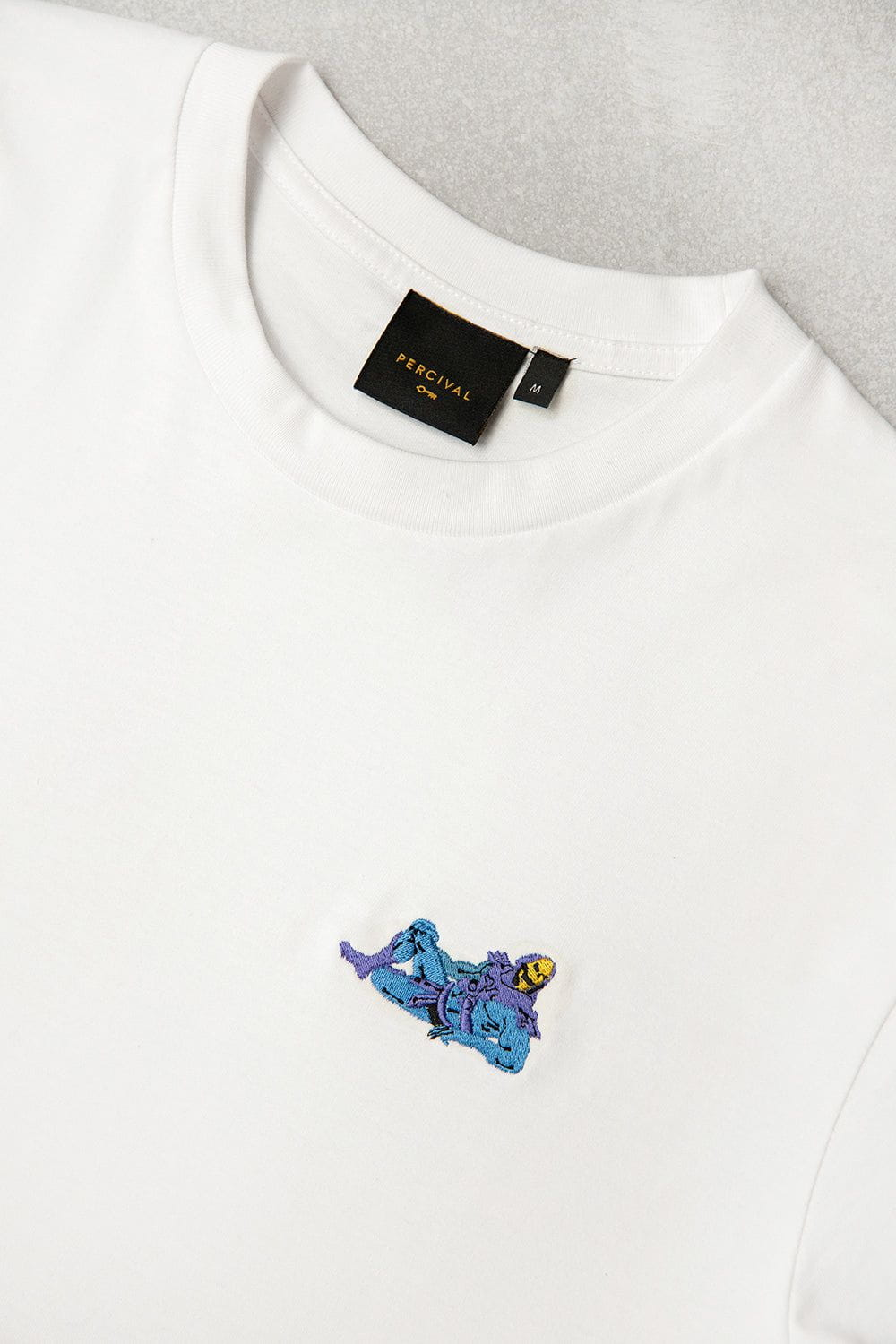• XS
• S
• M
• L
• XL
• XXL
• Select Size• XS
• S
• M
• L
• XL
• XXL
• Select Size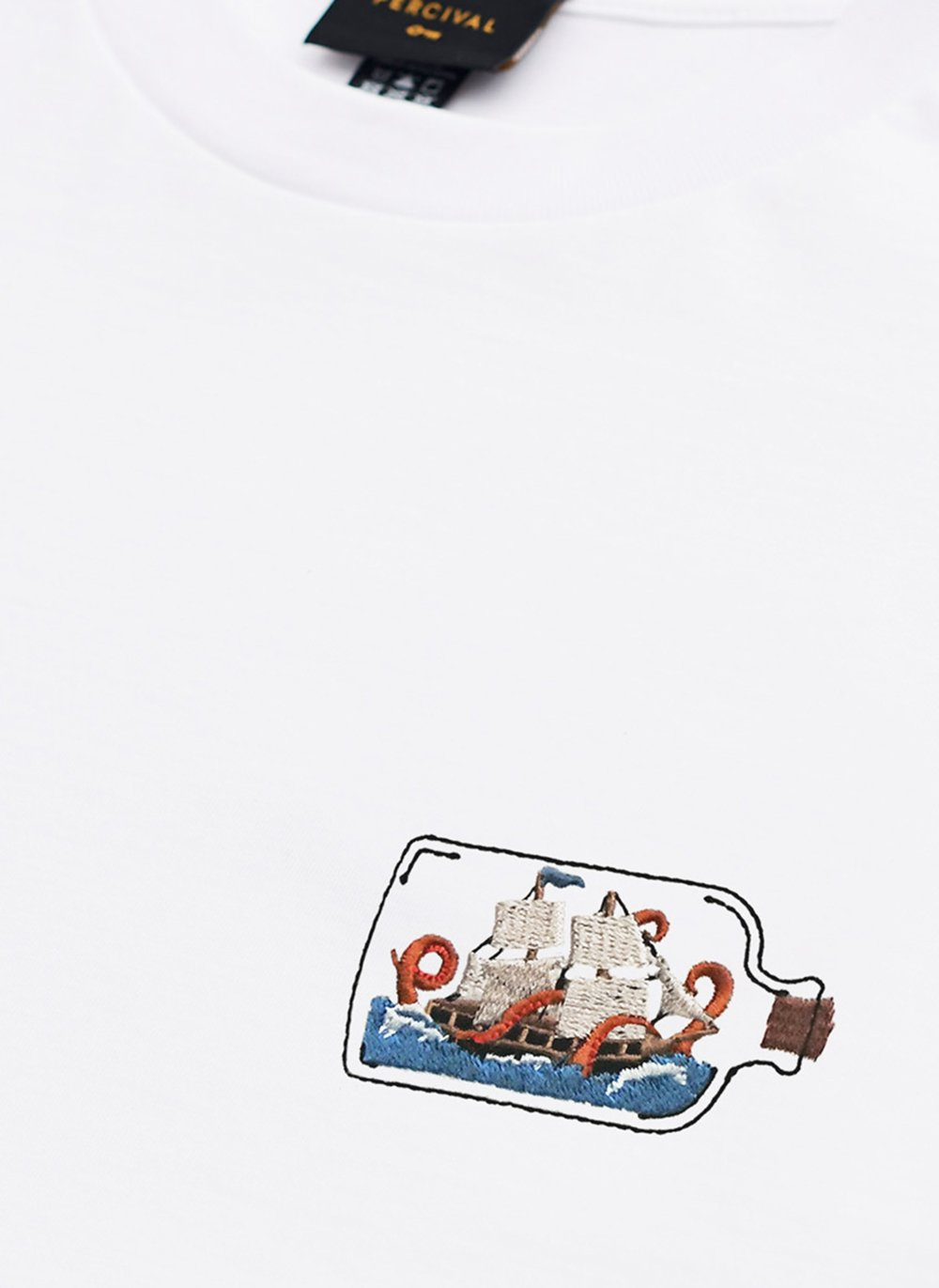• XS
• S
• M
• L
• XL
• XXL
• Select Size
Sold Out• XS
• S
• M
• L
• XL
• XXL
• Select Size• XS
• S
• M
• L
• XL
• XXL
• Select Size• XS
• S
• M
• L
• XL
• XXL
• Select Size• XS
• S
• M
• L
• XL
• XXL
• Select Size